9 篇文章 0 订阅

操作符概览

null合并??

*越靠上的操作符运算优先级越高,当同行操作符组成表达式时,大多数情况下,由左至右运算(赋值操作符不同,它是从右至左)

操作符的本质

• 操作符的本质是函数(即算法)的简记法

• 假如没有发明 " + " 而只有Add函数,算数" 3+4+5"将写成

• 假如没有发明" x " 而只有Mul函数, 那么算数"3+4*5 " 将写成

• 操作符不能脱离与它关联的数据类型

int x = 3;
int y = 2;
int z = x / y;
Console.WriteLine(z);
// Z输出为1 这是因为这是整数类型里的除号,所以进行整除

double a = 3;
double b = 2;
double c = a / b;
Console.WriteLine(c)
//C将输出1.5 double是双精度浮点数,将进行浮点数运算

每一种数据类型都有一套与该类型相关的操作与运算

• 可以说操作符就是与固定数据类型相关联的一套基本算法的"简记法"
示例:
usr
class Program
{
static void Main(string[] args)
{
Person persion1 = new Person();
Person person2 = new Person();
persion1.Name = "lz";
person2.Name = "lz's wife";
List<Person> nation = persion1 + person2;
foreach (var p in nation)
{
Console.WriteLine(p.Name);
}
}

}
class Person
{
public string Name;
public static List<Person>operator +(Person p1,Person p2)
{
List<Person> people = new List<Person>();
for (int i = 0; i < 11; i++)
{
Person child = new Person();
child.Name = p1.Name + "&" + p2.Name + "s'fz";
}
return people;
}
}

操作符的优先级

1. 可以使用圆括号提高被括起来表达式的优先级
2. 圆括号可以嵌套
3. 不像数学里有方括号和花括号,在C#语言里"[]“与”{}"有专门的用途
• 同级操作符的运输顺序
1. 除了带有赋值功能的操作符,同优先级操作符都是由左向右进行运算
2. 带有赋值功能的操作符的运算顺序是由右向左
int a = 100;
int b = 200;
a = a + b;
Console.WriteLine(a); //可以看出先运行a+b 然后再给a赋值 所以最后输出a=300
int x = 1;
int y = 2;
int z = 3;
x += y += z;
Console.WriteLine(x); //最后运算x+=y 所以x值为6
Console.WriteLine(y); //先运算y+=z 所以输出y值为5
Console.WriteLine(z); // z输出为3
• 与数学运算不同,计算机语言的优先级运算没有结合率

各类操作符的示例

基本操作符

" x.y "运算符 (成员访问操作符)

1. 访问外层命名空间的子集命名空间
System.IO  //访问System命名空间中的IO命名空间
1. 访问名称空间当中的类型
IO.file  //访问名称空间中的类型
1. 访问类型当中的静态成员
File.Create("D:\\HelloWorld.txt"); //访问类型当中的静态成员
1. 访问对象中的成员
Form myForm = new Form();
myForm.Text = "Hello,World";
myFrom.ShowDialog();

" f(x) "方法调用操作符

1. 调用方法示例
class Program
{
static void Main(string[] args)
{
Calculator c = new Calculator();
Console.WriteLine(x);
c.PrintHello(); //()不能省略,哪怕他没有参数
}

}
class Calculator
{
public double Add(double a , double b)
{
return a + b;
}
public void PrintHello()
{
Console.WriteLine("Hello");
}
}

" a[x] " 元素访问操作符

1. 访问数组中的元素
int[] myIntArray = new int[] { 1, 2, 3, 4, 5 };
Console.WriteLine(myIntArray);
Console.WriteLine(myIntArray.Length);
1. 访问字典中的元素
static void Main(string[] args)
{
Dictionary<string, Student> stuDic = new Dictionary<string, Student>();
for (int i = 0; i < 100; i++)
{
Student stu = new Student();
stu.Name = "s_" + i.ToString();
stu.Score = 100 + i;
}
Student number6 = stuDic["s_6"];
Console.WriteLine(number6.Score);
}

}
class Student
{
public string Name;
public int Score;
}

" x++ " , " x–" 后置自增&后置自减

1. 自增&自减
int x = 100;
x++
Console.WriteLine(x) //x输出101
int y = 100;
y--
Console.WriteLine(y); //y输出99
1. *易错 自增自减与赋值
int x = 100;
int y = x++;
Console.WriteLine(x); //此时x输出101
Console.WriteLine(y); //此时y输出100
//当后置自增(自减)赋值时,先将未自增(自减)的值赋值给变量,然后再自增;
• 上面例子相当于
int x =100
int y = x ;
x++;
Console.WriteLine(x);
Console.WriteLine(y);

“new” 创建一个实例并调用

var

var : 声明一个隐式类型变量
C#是强类型语言,强类型语言要求每一个变量都拥有自己的数据类型 比如说 int x 这就是一个显式类型变量

var x = 100; //这里100后面什么都不加那么100就是整型,赋值给x,x就是整型了
Console.WriteLine(x.GetType().Name);//输出int32
var y = 100D; //这里100后面加了个D代表着这个100是double类型,赋值给y之后 y就是double类型;
Console.WriteLine(y.GetType().Name));//输出double
var z = "100"; //这里100用双引号括起来,代表这个100为字符串类型,赋值给z之后,z就是字符串类型;
Console.WriteLine(z.GetType().Name));//输出string

//因为c#是强类型语言,所以当一个你给一个隐式类型赋值了之后确定了它的值类型,那么就不可以再给它赋其他类型的值了如下
z = 100; //这里会报错,字符串不能转化成整数类型

new 操作符
new 主要功能

new Form(); //new操作符后面跟上一个类型,就是在内存空间中创建Form这个类型的实例,创建完了之后()调用它的实例构造器

From myFrom;//声明一个同类型的变量;
myFrom=new Form();//引用它在这里插入代码片
//从这里我们可以看出new这个操作符除了能在内存上创建它的实例并且调用它的实例构造器之外呢,
//还能得到这个实例的内存地址,并且把这个内存地址通过赋值符号交给负值访问这个实例的变量,
//这样就在变量和实例之间构成了引用关系,有了这个引用关系,我们就可以通过变量来访问实例.
myFrom.text = "Hello"; //现在可以更改实例的属性了
myFrom.ShowDialog();
new 次要功能

new 能调用实例的初始化器,就是在调用实例的构造器()之后加上一对花括号{},我们可以在这对花括号里立刻为实例初始化值,示例如下

From myFrom = new From() {Text="Hello" }; //在调用构造器之后调用初始化器,将Text值初始化为Hello
//也可以初始化多个属性 使用逗号 " , " 分割就好
myFrom.ShowDialog();
new 小知识
并不是所有的实例创建都需要使用new操作符

string name = "猪头";//编译器并没有报错,我们也一直是这样使用的
int age = 100; //和声明结构类型的int 语法一样

string a = new string("Hello");
Console.WriteLine(a);
//a打印出来的是Hello 可见 string a = new string("Hello") 与 string a = "Hello"并没什么两样

int[] myArray = new int;
//在这里,我们使用new创建int数组实例 但我们也可以不使用new创建
int[] myArrays = {1,2,3,4,5,6,7,8,9}
//这样也能创建int数组 也没有使用new从操作符
new为匿名类型创建对象

Form是一个对象,且有自己的名字

Form myForm = new Form(){Text="Hello"}; //这是我们对于new操作符的最常见使用方法
//是不是new后面必须要跟一个数据类型才能创建对象呢? 答案是否的

new 在创建实例对象的时候要跟数据类型,这是对于非匿名对象来说才需要对于匿名对象来说

new{Text="猪头",Age=100}; //这里我们在new操作符后面不声明类型,直接跟上一个初始化器并随意初始化了两个属性
//属性名不重要,可以自定义,
//在这里我们并没有告诉编译器,text,与age是什么数据类型,但是没关系编译器会自己推断
//比如说text,赋的值是"猪头"那么它就是string类型,age赋的值是100,那么他就是int类型

//var声明一个隐式类型变量
//也就是说你并不能提前知道var声明的是什么变量,=赋值给它什么类型的变量,那么它就是什么类型
//所有
var myVariable; //var声明的变量没有类型,你给它什么类型,它便是什么类型
myVariable=new{Text="猪头",Age=100}; //引用刚刚声明的对象

var myVariable = new{Text="猪头",Age=100};
Console.WriteLine(myVariable.Text);//输出猪头
Console.WriteLine(myVariable.Age);//输出100
//可以正常使用

var myVariable = new{Text="猪头",Age=100};
Console.WriteLine(myVariable.Get);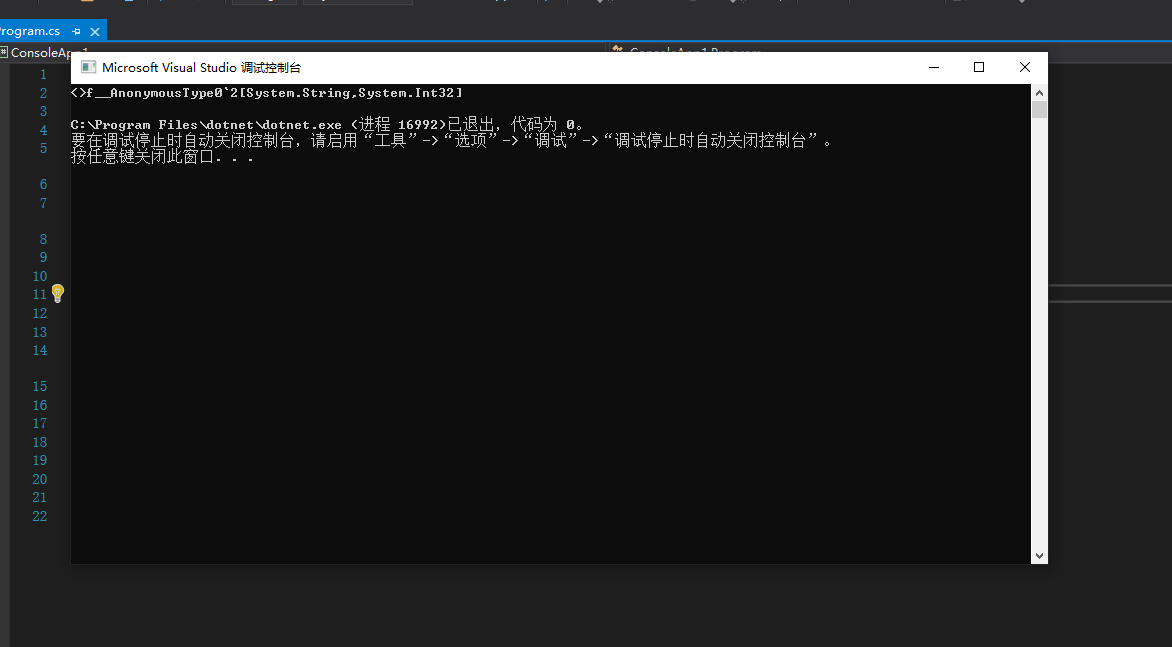如图可见,输入为<>f__AnonymousType0`2[System.String, System.Int32]
<>f__AnonymousType : 匿名类型前缀
0 : 我们在程序当中创建的第一个
'2:这是一个泛型类,构成这个类型需要两个类型
[System.String, System.Int32] : 构成的类型为 System.String, System.Int32

new注意事项

new操作符的功能非常强大也是一个非常重要的操作符,但功能越强大,却越容易滥用,后果也越难预料越难控制;比如你在一个类里面调用new操作符创建一个实例,那么你正在编写的这个类型,和你正在创建实例的这个类型之间就构成了非常紧密的耦合

class Program
{
static void Main(string[] args)
{
Form myForm = new Form() { Text = "猪头" };
//我在Program 这个类里面创建了Form这个类类型的实例
//这个时候Program 与Form就紧紧的耦合在了一起
//当Form这个类出错的时候,Program也就没办法运行了
}
//所以在使用new的时候要慎用
}
new 关键字(额外内容)
class Program
{
static void Main(string[] args)
{
var myClass = new IsClass();
myClass.Report();
var myClasss = new Classs();
myClasss.Report();
}

}
class IsClass
{
public void Report()
{
Console.WriteLine("I'm a Class");
}
}
class Classs:IsClass //继承IsClass类
{
new public void Report() //子类对父类方法的隐藏
{
Console.WriteLine("I'm Classs");
}
}

static void Main(string[] args)
{
Type t = typeof(int);
Console.WriteLine(t.Namespace);//查看命名空间
Console.WriteLine(t.FullName);//全名
Console.WriteLine(t.Name);//名称
int c = t.GetMethods().Length;
foreach (var mi in t.GetMethods())
{
Console.WriteLine(mi.Name);
}
Console.WriteLine(c); //查看有多少方法
}

"default " 取类型默认值

1. 结构体类型(值类型)
double x = default(double);
Console.WriteLine(x);
//x返回值为0
1. 引用类型(与值类型相对)

1. 枚举类型(值类型)
声明一个名为Level的枚举类型并查看它的default
class Program
{
static void Main(string[] args)
{
Level level = default(Level);
Console.WriteLine(level);
//返回Low,
这是因为在你声明枚举类型的时候,编译器会将它和整数值对应起来,第一个为零,往后依次加一
}

}
enum Level
{
Low,
Mid,
High
}

class Program
{
static void Main(string[] args)
{
Level level = default(Level);
Console.WriteLine(level);
//这里输出的值为Mid/因为我已经为Mid赋值为0
}

}
enum Level
{
Low=3,
Mid=0,
High=1
}

class Program
{
static void Main(string[] args)
{
Level level = default(Level);
Console.WriteLine(level);
//这里不会输出任何一个枚举属性,因为没有任何一个枚举属性值为零,所以,这里单单输出一个参数0
}

}
enum Level
{
Low=3,
Mid=2,
High=1
}

“checked” "uncheckecd"检查值是否有溢出&不检查值是否溢出

checked检查值是否有溢出

//uint:无符号整型
//占四个字节(32个bit)来表示它的数值,也就是说它能表示0至2的32次方-1
uint x = uint.MaxValue; //获取到 uint能表示的最大值
Console.WriteLine(x); //最大值为4294967295
string binStr = Convert.ToString(x,2);//查看它的二进制表示
Console.WriteLine(binStr);//表示为:11111111111111111111111111111111
uint y = x + 1; //这个时候我们给x加一
//因为x已经是uint能表示的最大值了,那么再加一显然是不行的
Console.WriteLine(y);//打印出y看看有什么
//0这是y所打印出来的值

uint y =checked( x + 1);
Console.WriteLine(y);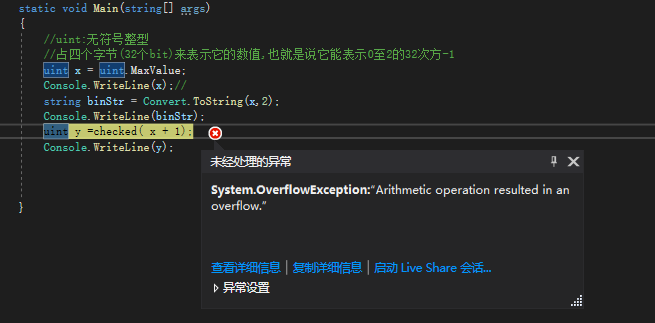static void Main(string[] args)
{
//uint:无符号整型
//占四个字节(32个bit)来表示它的数值,也就是说它能表示0至2的32次方-1
uint x = uint.MaxValue;
Console.WriteLine(x);//
string binStr = Convert.ToString(x,2);
Console.WriteLine(binStr);

try
{
uint y = checked(x + 1);
Console.WriteLine(y);
}
catch (OverflowException e)
{

Console.WriteLine("There‘s overflow"); ;
}
}
uncheckecd不检查值是否溢出(c#默认)

static void Main(string[] args)
{
//uint:无符号整型
//占四个字节(32个bit)来表示它的数值,也就是说它能表示0至2的32次方-1
uint x = uint.MaxValue;
Console.WriteLine(x);//
string binStr = Convert.ToString(x,2);
Console.WriteLine(binStr);

try
{
uint y = unchecked(x + 1);//将checked改为unchecked,编译器将不报错
Console.WriteLine(y);
}
catch (OverflowException e)
{

Console.WriteLine("There‘s overflow"); ;
}

}
checked&uncheckecd作为关键字使用

checked&uncheckecd作为关键字使用能检查一段代码块的值溢出 示例如下

static void Main(string[] args)
{
//uint:无符号整型
//占四个字节(32个bit)来表示它的数值,也就是说它能表示0至2的32次方-1
uint x = uint.MaxValue;
Console.WriteLine(x);//
string binStr = Convert.ToString(x,2);
Console.WriteLine(binStr);

checked //checked作为关键字使用能够检查它后面花括号括起来的的代码块是否有值溢出
{
try
{
uint y = x + 1;
Console.WriteLine(y);
}
catch (OverflowException e)
{

Console.WriteLine("There‘s overflow"); ;
}
}

}

unchecked使用方法同理

“delegate”

<Grid>
<Grid.RowDefinitions>
<RowDefinition Height="Auto"></RowDefinition>
<RowDefinition Height="2"></RowDefinition>
<RowDefinition Height="Auto"></RowDefinition>
</Grid.RowDefinitions>
<TextBox x:Name="myTextBox" Grid.Row="0"></TextBox>
<Button x:Name="myButto" Content="Click Me!" Grid.Row="2"></Button>
</Grid>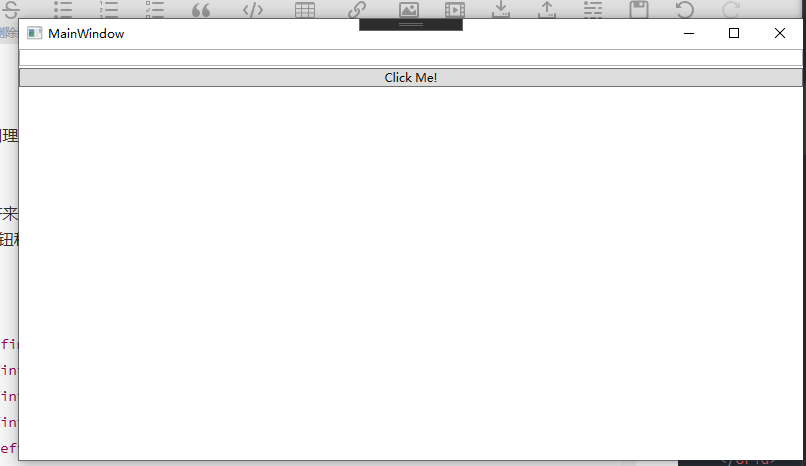再为button添加一个事件处理器

public MainWindow()
{
InitializeComponent();
this.myButton.Click += MyButton_Click; //通过+=挂接上一个事件处理器
}

private void MyButton_Click(object sender, RoutedEventArgs e)
{
this.myTextBox.Text = "Hello,World!";
}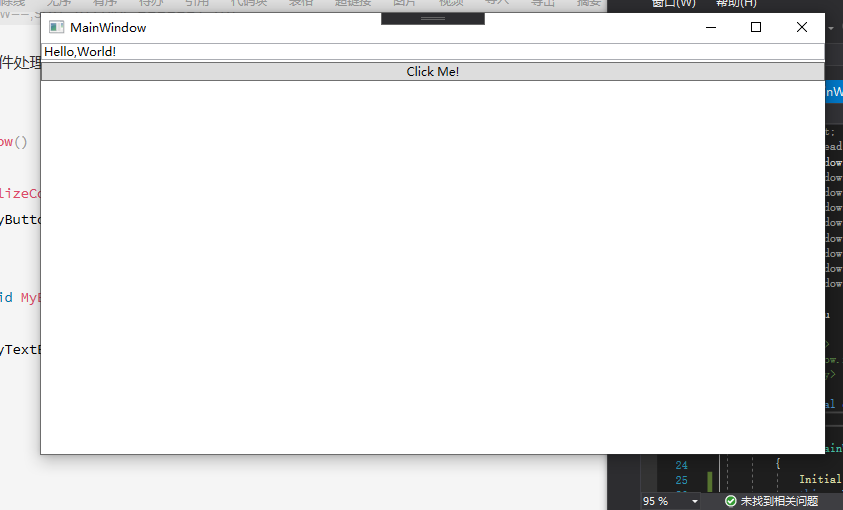我们为什么需要方法,或者函数呢?:是因为这个方法或者函数它封装了一定逻辑,可以在其他的地方得到重用,如果我们发现我写的这个逻辑永远不会在其他的地方被重用,我们也就可以不将它写成方法,我们可以使用匿名方法的方法,将方法的名字删掉,返回值也删掉,他就变成匿名方法了,然后将它放在原先挂接函数名称的地方,函数并在前方加上delegate如下图:

public MainWindow()
{
InitializeComponent();
this.myButton.Click += delegate (object sender, RoutedEventArgs e)
{
this.myTextBox.Text = "Hello,World!";
};
}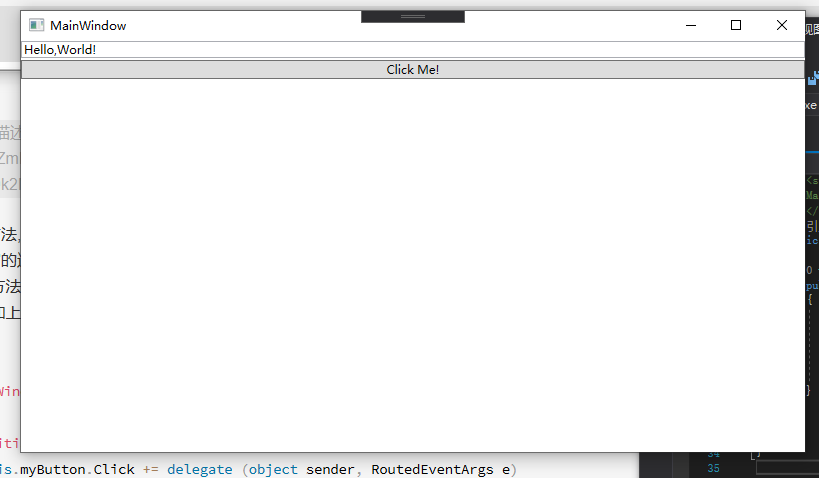在前面说过,这种方法已经过时了,因为我们有拉姆达表达式,拉姆达表达式,比隐式方法还要简洁

public MainWindow()
{
InitializeComponent();
this.myButton.Click += (sender, e) =>
{
this.myTextBox.Text = "Hello,World!";
};
}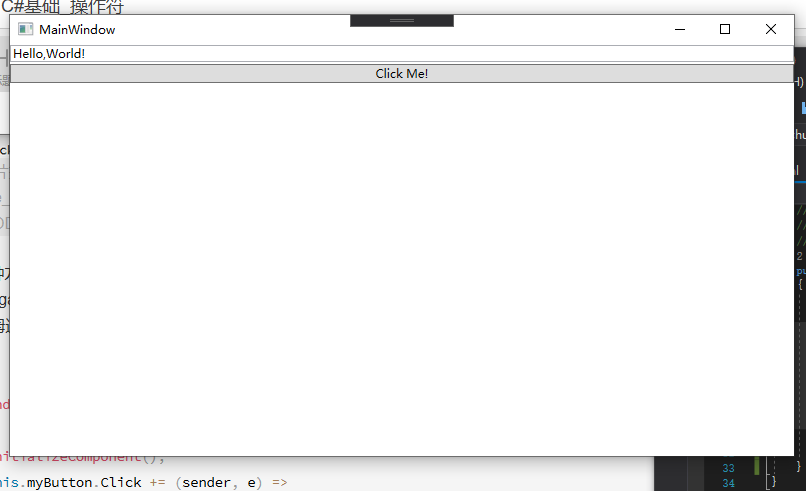从有名字的函数/方法进化成没有名字的匿名方法,再到现在的拉姆达表达式,C#越来越简洁

"sizeof"获取一个对象在内存中所占字节数

sizeof注意事项
1. 默认情况下sizeof只能用于获取基本数据类型的示例在内存中所占的字节
基本数据类型除string和object的所有c#关键字的数据类型,因为sizeof只能用于获取结构体数据类型在内存中占的字节数.string和object不是结构体
默认情况下示例
int a = sizeof(int); //查看int所占字节
Console.WriteLine(a); //占四个字节
int b = sizeof(double); //查看double占多少个字节
Console.WriteLine(b);//占八个字节
int c = sizeof(uint);//查看uint占多少个字节
Console.WriteLine(c);//占四个字节
int d = sizeof(decimal);//查看decimal占多少个字节
Console.WriteLine(d);//占一十六个字节
1. 在非默认情况下我们可以使用sizeof获取自定义的结构体类型的实例在内存中占的字节数但是需要将它放在不安全的上下文当中
非默认情况下示例:
class Program
{
static void Main(string[] args)
{
unsafe //编译器编译不过去,需要将代码放在unsafe代码块中,并将项目属性调整为允许unsafe
//这样我们就能查看自定义实例对象在内存中所占字节数了
{
int x = sizeof(Student);//查看该实例所占字节
Console.WriteLine(x);
}
}
}
class Student //自定义一个类
{
int ID;
string Name;
}

“->” 指针

-> 用于直接操作内存,需要放在不安全的代码块内才能运行,指针只能用于操作结构体类型,不能用于操作引用类型,示例如下

class Program
{
static void Main(string[] args)
{
unsafe
{
Student stu;
stu.ID = 1;
stu.Score = 99;
Student* pStu = &stu;
pStu->Score = 100;
Console.WriteLine(stu.Score);

}

}

}
class Student
{
public int ID;
public long Score;
}

一元操作符(单目操作符)

“+” “-” “~” 取正&取负&取反

“+” “-“与数学运算符”+” "-"一致的地方
int a = 100; //声明一个int a 并赋值100
int b = +a; //取a的正数
Console.WriteLine(b); //输出为100
int c = -a;//取a的负数
Console.WriteLine(c);//输出为-100
int d = --a;//取负再取负
Console.WriteLine(d);//输出为99,因为--是前置自减
int e = -(-a);//取负再取负
Console.WriteLine(e);//输出为100
“+” “-” “!”

Console.WriteLine(int.MaxValue);//int最大值:为2147483647
Console.WriteLine(int.MinValue);//int最小值为-2147483648

//从常识上来讲int既然能装下它的最小值,那也应该能装下它最小值的绝对值
int x = int.MinValue; //获取int最小值
int y = -x; //取负,并赋值给y
Console.WriteLine(x); //打印出x 值为-2147483648;
Console.WriteLine(y); //打印出y 值为-2147483648;

int x = int.MinValue;
int y = checked( -x);//运行不下去,编译器会报错System.OverflowException:“Arithmetic operation resulted in an overflow.”
//这个值溢出了
Console.WriteLine(x);
Console.WriteLine(y);

~,求反操作符,功能是在一个结构数在二进制级别上进行按位取反(意思就是,0就变为1,1就变为0,二进制)

int x = 12345678;
int y =~x;
Console.WriteLine(y);//y打印值为:-12345679
//这是为什么呢?,我们可以再来看看y和x二进制值
string xStr = Convert.ToString(x, 2).PadLeft(32, '0');
string yStr = Convert.ToString(y, 2).PadLeft(32, '0');
Console.WriteLine(xStr);//打印值为:00000000101111000110000101001110
Console.WriteLine(yStr);//打印值为:11111111010000111001111010110001
//可以看出,在y是x按位取反的数

int x = int.MinValue; //获得int最小值
int y = -x;//取反
Console.WriteLine(y);//值输出=x
string xStr = Convert.ToString(x, 2).PadLeft(32, '0');//查看x的二进制
// x的值为10000000000000000000000000000000
//按位取反01111111111111111111111111111111
//根据规则,取反等于按位取反加一,所以
//取反值为10000000000000000000000000000000
//所以y值输出为x
Console.WriteLine(xStr);

“!” 取非

bool b1 = true;
bool b2 = !b1; //取非
Console.WriteLine(b2) //输出False

class Program
{
static void Main(string[] args)
{
Student stu = new Student(null);
Console.WriteLine(stu.Name);
}
}
class Student
{
public Student(string initName)
{
if (!string.IsNullOrEmpty(initName))
{
this.Name = initName;
}
else
{
throw new ArgumentException("inittName cannot be null or empty");
}
}
public string Name;

}

“++x” “–x”,前置自增,前置自减

int x = 100;
int x1 = x++; //后置自增
int x2 = ++x;//前置自增
int x3 = x--;//后置自减
int x4 = --x;//前置自减
Console.WriteLine(x1);//输出值为101
Console.WriteLine(x2);//输出值为101
Console.WriteLine(x3);//输出值为99
Console.WriteLine(x4);//输出值为99

int x = 100;
int y = x++;//当后置自增前面是赋值符号时,会原先将自己的值赋值给变量,然后再自增
Console.WriteLine(x); //输出值为101
Console.WriteLine(y);//输出值为100

int x = 100;
int y = --x;//当前置自增(自减)前面是赋值符号时,会先自增(自减)后再将值赋值给变量
Console.WriteLine(x); //输出值为101
Console.WriteLine(y);//输出值为101

"<T>x"强制类型转换

<T>x强制类型在转换看这类型转换

Console.WriteLine(ushort.MaxValue);//查看ushort类型的最大值,其值为65535
uint x = 65536; //给类型为uint的变量赋值为65536(比ushort所能存储的最大值大一)
/*
ushort y=x //这里这样直接赋值不行,会出错我们需要cast强制将x转换成ushort然后赋值给y
*/
ushort y = (ushort)x;//使用cast,即(T)x的方式强制将x转换为ushort类型,然后赋值给y
Console.WriteLine(y);//输出y,值为零

算数运算操作符

1. 任何一个操作符都是与它操作的数据类型相关的,也就是说当它操作的数据类型不同,会有不同的运算规则
示例下:
int x = 1; //int数据类型
int y = 2;//int数据类型
int z = x + y; //int数据类型加int数据类型
Console.WriteLine(z); //输出值为两个变量相加.结果为3
string a = "1";//string类型
string b = a + y;//string类型加int类型
Console.WriteLine(b);//输出值为两个字符串拼接.结果为12

2.C#中有取余这个操作符,在数学运算当中是没有的

int x = 3; ;
int y = 4;
int z = x%y; //取余
Console.WriteLine(z);//余数为3

C#算数运算中的数值提升

int a = 3;
double b = 4;
//乘法
var c = a * b;
//除法
var d = a / b;
//求余
var e = a % b;
//加
var f = a + b;
//减
var g = a - b;
Console.WriteLine(c.GetType().FullName);//输出double
Console.WriteLine(d.GetType().FullName);//输出double
Console.WriteLine(e.GetType().FullName);//输出double
Console.WriteLine(f.GetType().FullName);//输出double
Console.WriteLine(g.GetType().FullName);//输出double

int x = 100;
uint y = 200;
var z = x + y;
Console.WriteLine(z.GetType().FullName);//输出int64,也就是长整型long

long x = 100;
ulong y = 200;
var z = x + y;//报错运算符"+"对于long和ulong类型的操作具有二义性
Console.WriteLine(z.GetType().FullName);

var x = (double)5 / 4;
var y = (double)(5 / 4);
var z = 5 / (double)4;
Console.WriteLine(x);//输出1.25 //进行浮点除法运算
Console.WriteLine(y);//输出1		//进行整数除法运算
Console.WriteLine(z);//输出1.25	//进行浮点除法运算

“*” “/” “%” 乘法,除法,求余

乘法

整数乘法

int operator *(int x, int y)
uint operator *(uint x, uint y);
long operator *(long x, long y);
ulong operator *(ulong x, ulong y);

unchecked
{
//这里就不使用checked来检测溢出了我们知道int.MaxValue*5是肯定溢出的,我们来看它溢出的是什么
int x = int.MaxValue;//获取intMax值
int y = 5;
int intZ = x * y;//使用int类型接收这个超出int最大结果范围的数
string stringIntZ = Convert.ToString(intZ, 2).PadLeft(64, '0');//查看这个数的二进制,不足64位使用0补足
long longZ = (long)x * (long)y;//使用long接收int最大值乘2,long是长整型64位肯定能接收下这个数
Console.WriteLine(intZ); //输出2147483643
Console.WriteLine(longZ);//输出10737418235
Console.WriteLine(stringIntZ); //输出//0000000000000000000000000000000001111111111111111111111111111011
Console.WriteLine(stringLongZ);//输出//0000000000000000000000000000001001111111111111111111111111111011
Console.WriteLine(Convert.ToString(intZ,2));//输出                      1111111111111111111111111111011
//为了更直观,我这里输出一个没有使用0补足的intZ的二进制
}

浮点乘法

float operator *(float x, float y);
double operator *(double x, double y);

+y–y+0–0+∞–∞NaN
+x+z–z+0–0+∞–∞NaN
–x–z+z–0+0–∞+∞NaN
+0+0–0+0–0NaNNaNNaN
–0–0+0–0+0NaNNaNNaN
+∞+∞–∞NaNNaN+∞–∞NaN
–∞–∞+∞NaNNaN–∞+∞NaN
NaNNaNNaNNaNNaNNaNNaNNaN
小数乘法

decimal operator *(decimal x, decimal y);

除法运算

整数除法

int operator /(int x, int y);
uint operator /(uint x, uint y);
long operator /(long x, long y);
ulong operator /(ulong x, ulong y);

int x = 5;
int y = 0;
int z = x / y;
Console.WriteLine(z);
//未经处理异常System.DivideByZeroException
//HResult=0x80020012
//Message=Attempted to divide by zero.
//Source=ConsoleApp1
//StackTrace:
//at ConsoleApp1.Program.Main(String[] args) in E:\ProgramData\Visual Studio\ConsoleApp1\ConsoleApp1\Program.cs:line 13

浮点除法

float operator /(float x, float y);
double operator /(double x, double y);

double x = 5;
double y = 0;
double z = x / y;
Console.WriteLine(z);//输出正无穷大
double a = double.PositiveInfinity;//正无穷大
double b = double.NegativeInfinity;//负无穷大
double c = a / b;
Console.WriteLine(c);//输出NaN
+y–y+0–0+∞–∞NaN
+x+z–z+∞–∞+0–0NaN
–x–z+z–∞+∞–0+0NaN
+0+0–0NaNNaN+0–0NaN
–0–0+0NaNNaN–0+0NaN
+∞+∞–∞+∞–∞NaNNaNNaN
–∞–∞+∞–∞+∞NaNNaNNaN
NaNNaNNaNNaNNaNNaNNaNNaN
小数除法

decimal operator /(decimal x, decimal y);

余数运算符

整数余数

int operator %(int x, int y);
uint operator %(uint x, uint y);
long operator %(long x, long y);
ulong operator %(ulong x, ulong y);
x % y 的结果是由 x – (x / y) * y 生成的值。如果 y 为零，则将引发 System.DivideByZeroException。

浮点余数

float operator %(float x, float y);
double operator %(double x, double y);

+y–y+0–0+∞–∞NaN
+x+z+zNaNNaNxxNaN
–x–z–zNaNNaN–x–xNaN
+0+0+0NaNNaN+0+0NaN
–0–0–0NaNNaN–0–0NaN
+∞NaNNaNNaNNaNNaNNaNNaN
–∞NaNNaNNaNNaNNaNNaNNaN
NaNNaNNaNNaNNaNNaNNaNNaN
小数余数

decimal operator %(decimal x, decimal y);

加法运算符

整数加法

int operator +(int x, int y);
uint operator +(uint x, uint y);
long operator +(long x, long y);
ulong operator +(ulong x, ulong y);

浮点加法

float operator +(float x, float y);
double operator +(double x, double y);

位移操作符

“<<” ">>"左右位移操作符

int x = 9;
int y = x << 2;//使用位移操作符将x的二进制数据向左位移两位
Console.WriteLine(strX);//输出"00000000000000000000000000001001"
Console.WriteLine(strY);//输出"00000000000000000000000000100100"

y = x << z;
x位需要位移的数值
z为x需要位移的位数
y等于x乘2的z次幂
(>>右移符号反之)
y = x ∗ 2 z y=x*2^{z}

关系和类型检测操作符

“>” “>=” “<” “<=” “=” “==”

int x = 5;
double y = 6;
var z = x >= y;//比较x是否大于等于y
Console.WriteLine(z.GetType().FullName);//输出bool
Console.WriteLine(z);//false

char a = 'a';
char b = 'A';
var c = a > b;
Console.WriteLine(c);//输出True

char a = 'a';
char b = 'A';
var c = a > b;
Console.WriteLine(c);
ushort ua = (ushort)a;
ushort ub = (ushort)b;
Console.WriteLine(ua);//输出97
Console.WriteLine(ub);//输出65

string strA = "abc";
string strB = "ABC";
var c = strA == strB;
Console.WriteLine(c);//输出False///因为字符大小不一样

"is"操作符

class Program
{
static void Main(string[] args)
{
Son s = new Son();
var result = s is Son; //这里所检验的不是这个变量,而是这个变量所引用的实例
Console.WriteLine(result);//输出true
//检验看看是不是说 is所检验的不是引用变量的类型而是引用变量的实例
Son s1 = null;//给s1 null
var result1 = s1 is Son;//检验
Console.WriteLine(result1);//输出flase 说明is所检验的不是引用变量的类型
var result2 = s is Father;//我们试试输入它的父类
Console.WriteLine(result2);//输出true,这就好比说,你是一个男人,你也是一个人,这没毛病老铁

}
}
class Grandfather//创建一个基类
{
public void Grandfathers()
{
Console.WriteLine("这是Grandfather");
}
}
class Father : Grandfather//继承Father
{
public void Fathers()
{
Console.WriteLine("这是Father");
}
}
class Son : Father//继承Father
{

public void Sons()
{
Console.WriteLine("这是Son");
}
}

“as”

class Program
{
static void Main(string[] args)
{
object o = new Son();//给引用变量o引用son实例
Son s = o as Son;//o判断是不是和son一样的,如果是就将son实例引用地址给s
if (s !=null)
{
s.Sons();
}
}
}
class Grandfather//创建一个基类
{
public void Grandfathers()
{
Console.WriteLine("这是Grandfather");
}
}
class Father : Grandfather//继承Father
{
public void Fathers()
{
Console.WriteLine("这是Father");
}
}
class Son : Father//继承Father
{

public void Sons()
{
Console.WriteLine("这是Son");
}
}

int x = 5;
int y = 6;
int z = x & y;
int z1 = x ^ y;
int z2 = x | y;
//是非是
//是是非
//是非非
//是非是
//是是非
//非是是
//是非是
//是是非
//是是是

"&&"AND条件与 "||"OR条件或

&&条件与

&&需要注意的是它的短路规则

int x = 5;
int y = 4;
int z = 3;
if (z > y && z++ > 3)//z>y是false所以不通过,并且因为条件与存在短路规格所以
//当z>y为false时z++>3就不会再运行了
//&&需要两边条件都成立,当一边条件不成立,那么另一半也就没有再运行的必要了,所以这个时候z还是等于3并没有自增
{
Console.WriteLine("Hello.第一次Z的值是"+z);
}
else if(x > y && z++ > 3)//x>y为true继续z++>3,因为z++是先使用值再自增,所以这里是false,但z++运行了所以z现在的值是4
{
Console.WriteLine("Hello.第二次Z的值是" + z);
}
else if(x > y && z++ > 3)//x>y为true,z++>3为true(因为上面虽然没有通过,但z在比较了之后自增了所以这里z=4>3),运行完之后z再一次自增变为5
{
Console.WriteLine("Hello.第三次Z的值是" + z);//输出z的值5
}
else
{
Console.WriteLine("Hello.第四次Z的值是" + z);
}

|| 条件或

||也有类似的短路规则

int x = 5;
int y = 4;
int z = 3;

if (x > y || z++ > 3)//x>y为true因为是条件或所以后面的条件会被编译器短路,也就是说z++>3不会运行z也就还是3
{
if (z > y || z++ > 3)//z>y为false编译器继续运行z++>3因为上面z++>3没有运行所以z现在还是等于3而且后置自增是在值使用之后再加一,所以这里z++>为false,之后代买z++再自增,此时z值为四
{
Console.WriteLine("地一轮Z值为"+z);
}else if (z > y || z++ > 3)//z>y为false,z++>3为true z++之后再自增值为五
{
Console.WriteLine("第二轮Z值为"+z);//输出z,其值为5
}
}

“??” Null值合并操作符

int studentA = null //这是错误的

Nullable<int> studentA = null;//这个时候int值可以使用null值了
int? studentB = null;//也可以这样写,它们的意义是一样的

int? studentB = null;//也可以这样写,它们的意义是一样的
studentB=studentB??2//

"?:"条件操作符

int x = 80;
string str = string.Empty;
if (x >= 60)
{
str = "及格";
}
else
{
str = "不及格";
}
Console.WriteLine(str);

int x = 80;
string str = string.Empty;
str = x > 60 ? "及格" : "不及格";
Console.WriteLine(str);
//而且我们甚至可以更简洁一点,这是这种的一般用不上
int x = 80;
string str = x > 60 ? "及格" : "不及格";
Console.WriteLine(str);

赋值操作符

int x = 5;
x = x + 1;
int y = 5;
y += 1;
Console.WriteLine(x);//输出6
Console.WriteLine(y);//输出6

y+=1;就相当于y=y+1;

int x = 3;
int y = 4;
int z = 5;
int a = x += y *= z;
Console.WriteLine(a);//输出的值是二十三

09-19
07-1867

06-044117
03-28147
09-2478
12-02381
03-31101
05-041132
11-071958
04-1518
02-212227
08-0418
07-28344
02-091003
04-224605
06-28154
08-23576
05-221718JiuMeilove

¥2 ¥4 ¥6 ¥10 ¥20余额支付 (余额：-- )扫码支付获取中扫码支付点击重新获取扫码支付1.余额是钱包充值的虚拟货币，按照1:1的比例进行支付金额的抵扣。
2.余额无法直接购买下载，可以购买VIP、C币套餐、付费专栏及课程。余额充值